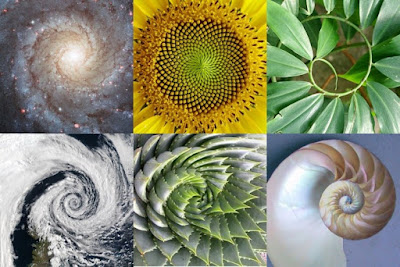April 06, 2018SPIRALS AND VORTEXES

The "secrets" to the creation of the Universe could be explained by many different aspects of life and science - Quantum Physics, Biology, Sacred Geometry, Sound, Mathematics, Human Chakras, etc. Vvortex-based mathematics could also explain Creation. It's called "vortex-based mathematics", because it is based on a vortex pattern. We can see vortexes everywhere - in our dna, in nature, plants, animals and even in the cosmos.If you're familiar with the Golden Ratio (phi) and how everything in life is trying to get closer to Phi, but never actually manages to do so, through the Fibonacci sequence, then you probably know better the importance of spirals and therefore - vortexes. Many people have already heard the Nikola Tesla's quote:
If you only knew the magnificence of the 3, 6 and 9, then you would have the key to the Universe.
In this article we will explain why exactly are 3, 6 and 9 so important.

THE ETERNAL ENERGY AND THE DUALITIES

We know that everything in the Universe is made out of energy. The term "energy" however is very complexed. We use this term in many different situations - to define the mass of a particle, to define someone's aura, to define our current phychological state, etc. In this article we will look at the term "energy" as the force from which everything is created - the Universe and every duality in it. This energy penetrates everything - every dimension, every living being, every planet, every star, etc. and it is constantly moving and it is eternal. As you know there are 9 single-digit numbers that exist - 1,2,3,4,5,6,7,8 and 9. Now, this symbol is referred as "The Symbol of Enlightenment" by Marko Rodin (the person that claimed to have tapped in this eternal energy and gave the knowledge regarding vortex-based mathematics).This symbol explains the duality (3 and 6) and the point of singularity from which all patterns in nature are created (9). Let's start by using doubling (the principle of cell division, which is also found in the computer's binary system).

1 doubled is 2
2 doubles is 4
4 doubled is 8
8 doubled is 16 = 1+6 = 7
7 doubled is 14 = 1+4 = 5
and 5 doubled is 10 = 1+0 = 1

So, as you can see, no matter how much you double the numbers they will always create this pattern which is infinite movement in a circular motion, changing polarities (3 and 6). Even if you do the doubling like so, you will still get the same pattern:

1 doubled = 2
2 doubled = 4
4 doubled = 8
8 doubled = 16 = 1+6 = 7
16 doubled = 32 = 5
32 doubled = 64 = 10 = 1
64 doubled = 128 = 11 = 2
128 doubled = 256  = 13  = 4
256 doubled = 512 = 8
512 doubled = 1024 = 7

No matter how much you double the numbers you will always get this pattern of 1,2,4,8,7,5 and back to 1 again. It is interesting to consider that when the pattern does 1 full cycle, it goes back to 1 by reaching the number 64 (6+4). In the cell division, once the number reaches 64 (stem cells), they start to become individual cells (brain cells, photoreceptor cells, heart cells, etc.)
If we go clockwise by doubling then that means we should be able to go counterclockwise by halving.

half of 1  = 0,5 = 5
half of 0,5 = 0,25 = 7
half of 0,25 = 0,125 = 8
half of 0,125 = 0,0625 = 13 = 4
half of 0,0625 = 0,03125 = 11 = 2
half of 0,03125 = 0,015625 = 19 = 10 = 1

But how come even though the constant change between the two opposites ( 3 and 6 ) is occuring, they are not connected by line, but are both connected to the number 9?

Let's start by doubling 3.
3 doubled = 6
6 doubled = 3 (12)
12 doubled = 6 (24)
24 doubled = 3 (48=13)

As you can see whether you start doubling 6 or 3 you will always get stuck in a constant change between each other. The same goes with halving as well.

3 halved = 6 (1,5)
1,5 halved = 3 (0,75 = 12)
0,75 halved = 6 (0,375 = 15)

But what happens if you double or halve the 9?

9 doubled is = 9 (18)
18 doubled = 9 (36)
36 doubled = 9 (72)
72 doubled = 9 (144)

9 halved = 9 (4,5)
4,5 halved = 9 (2,25)
2,25 halved = 9 (1,125)

The 9 is always the king of the system that gives the motion (vibrations) of the dualistic energies (positive and negative). Both 3 or 6 have to go through the 9. You can also see that if you combine the numbers that are in a line from the opposite sides of the picture above you will always get 9.8 + 1 = 9
7 + 2 = 9
6 + 3 = 9
5 + 4 = 9

9 polarizes and turns the numbers into mirror images. This can be easily illustrated by using multiplication tables. This symbol can do all functions of math (addition, subtraction, division, multiplication) simultaneously and instantaneously and always get perfect interaction between the polarized numbers. It can also model every principle of physics, the periodic table in chemistry, etc.

If you wish to learn more details regarding the perfection of this model, you can watch the following video or check the works of Marko Rodin and Randy Powell.